# 8+ Sample Metric Weight Conversion Chart Templates

When we speak of the units of weight, we instantly think of kilogram or gram. However, there are other units like milligram or even centigram or ton or quintal. Now, in our day to day lives, we are often required to convert between these different units. Unless you are exceptionally strong in mental calculations, it is always better to try out Metric Conversion Chart Templates that can help you switch between the various units as per your requirements.

## Simple Metric Conversion Weight Chart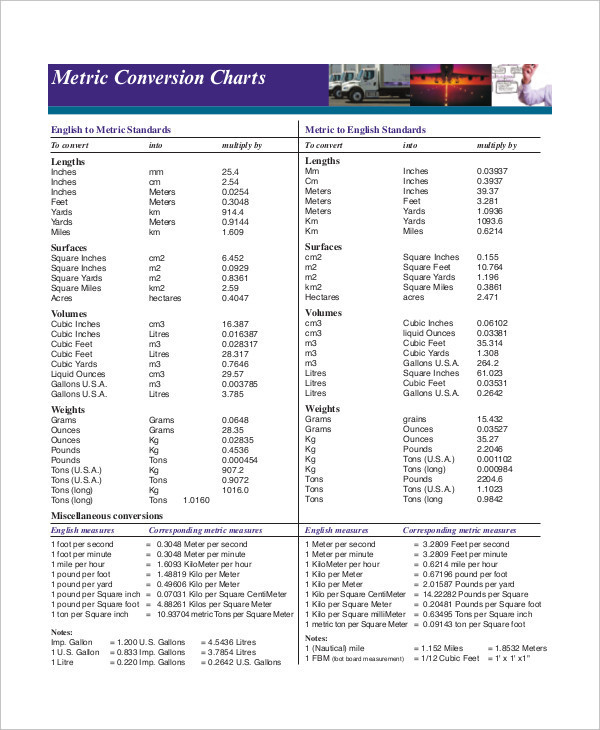stevensglobal.com

The simple weight conversion Chart Templates can help you convert from units in the International System of Units and the metric system. You can efficiently carry out important calculations here.

## Metric Height and Weight Conversion Chart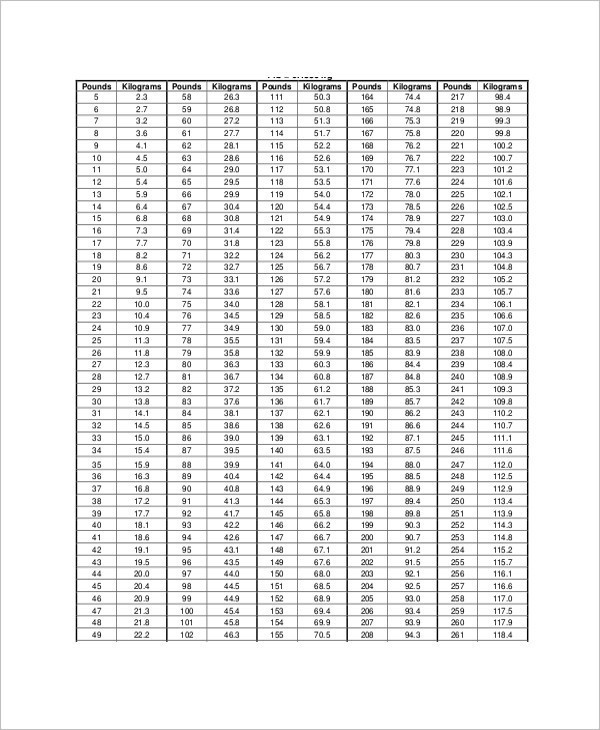calgarylabservices.com

This metric height and weight conversion chart contains the units of height like meter, centimeter, millimeter, inches and so on and the units of weight like gram, kilogram, ton, milligram or centigram.

## Metric System Weight Conversion Chart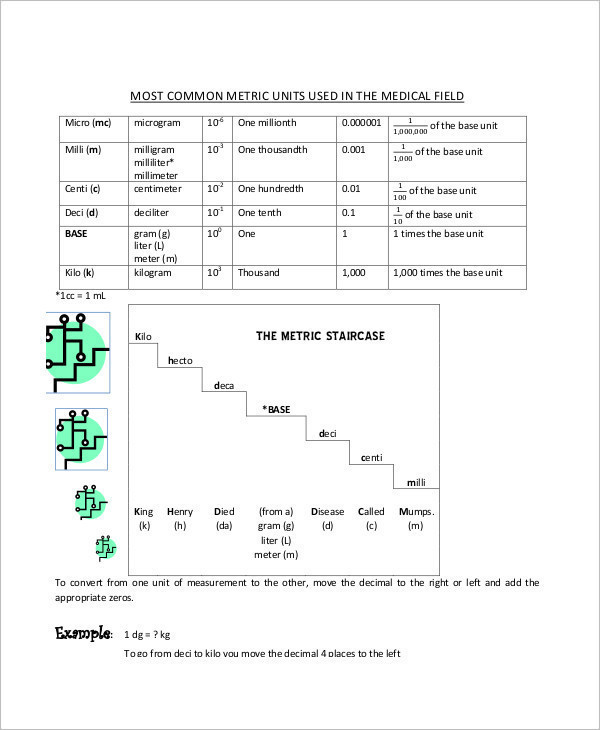laniertech.edu

This metric system weight conversion chart allows you to seamlessly convert between the different units of weight in a simple and effortless manner. Also, the calculations are made simpler.

## Metric Weight Conversion Table Chart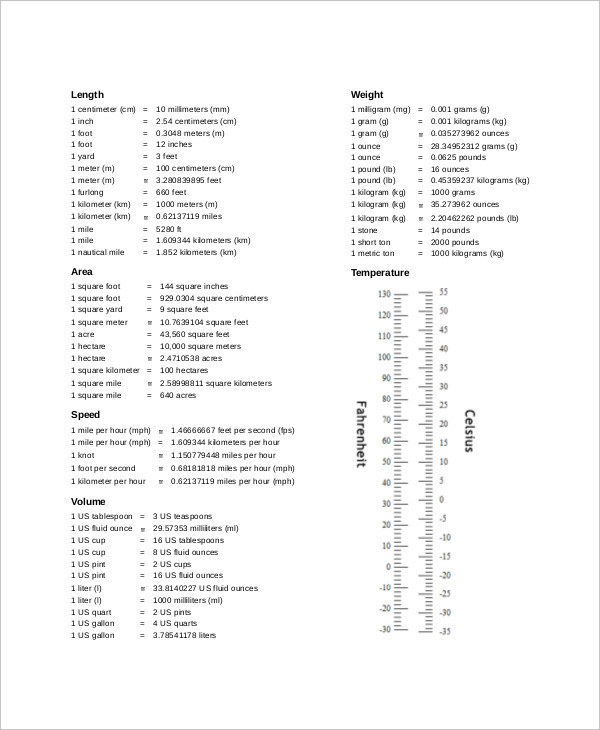quizzes.cc

When it comes to mathematical conversions, tables are always more convenient. That is because the metric conversion table charts are more organized and specific. Also, they are very easy to use.

## Metric Unit Weight Conversion Chart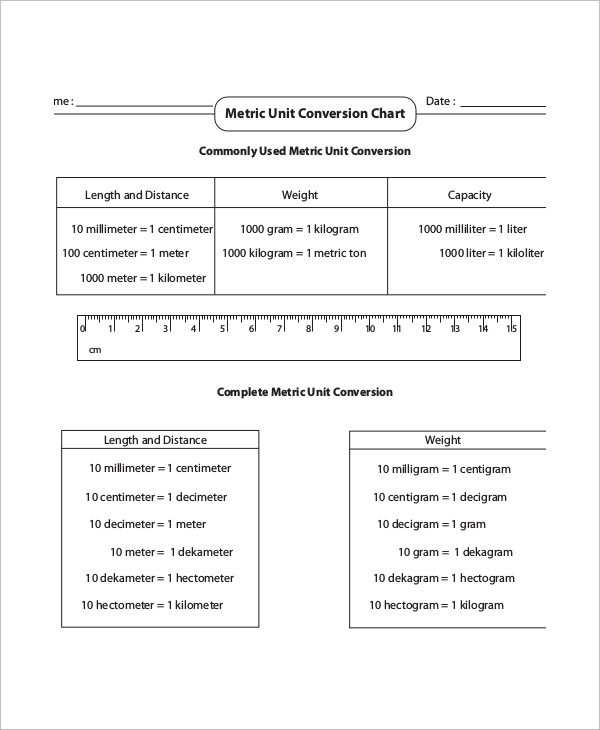mathworksheets4kids.com

## Sample Imperial Metric Weight Conversion Chart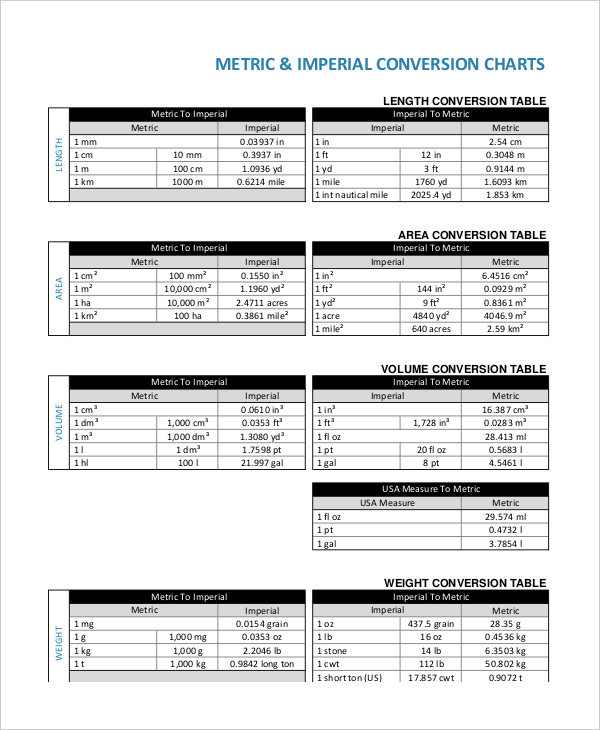championfreight.co.nz

## Metric Measurement Weight Conversion Chart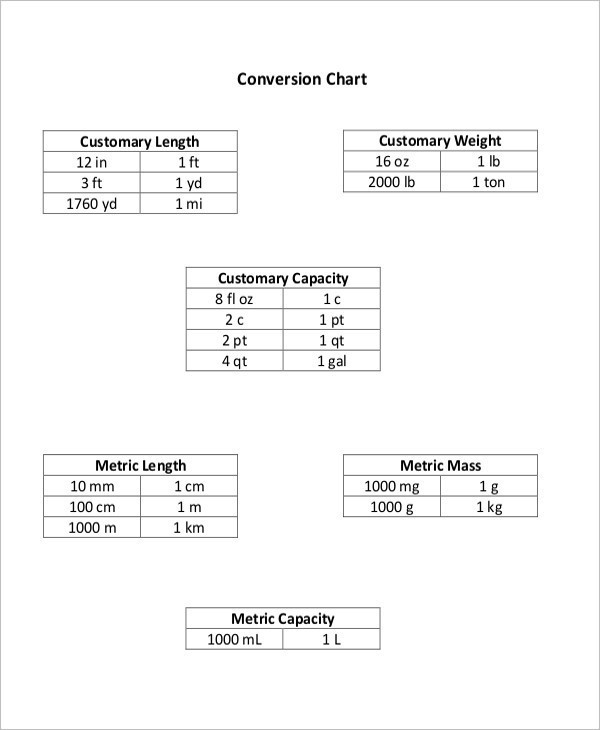wccusd.net

## Metric Mass Weight Conversion Chart Example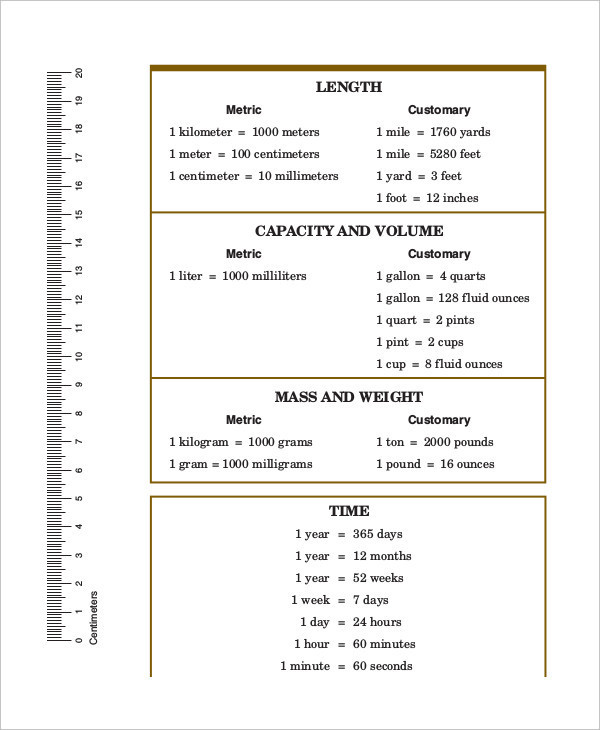tarleton.edu

## English to Metric Weight Conversion Chart

In case you are planning to convert the units of weight from English to the metric system, you would find this English to metric weight conversion chart to be exceptionally helpful.

### > Why Should you use the Metric Weight Conversion Charts?

In the International System of Units, the unit of weight is kilogram. However, in our everyday lives, we usually use the units of gram, milligram and centigram to express our weight. Similarly, when we speak of large scale items, kilogram as a unit will not suffice. You can also see Metric System Conversion Chart Templates.

Bigger units like ton or quintal would be more appropriate. That means you need to know the connection between each and every unit to convert between them accurately. To make it more convenient for you, there are weight conversion charts which can help out with the calculations and would even give you accurate results.

### > What are the benefits of the Metric Weight Conversion Charts?

The metric weight conversion charts that you see here come with the following benefits:

• The charts contain all the units of weight you can think of. Thus, if you are required to deal with some obscure units, you wouldn’t have to worry about it.
• The charts can be used any number of times. Thus, if you are required to deal with such conversions on a daily basis at your place of work or in school, you could use the weight conversion charts.
• The charts are really easy to use. Even children in primary school would be able to use the charts with ease. You can also see Simple Metric Conversion Chart Templates.

The next time you are required to carry out complicated conversions between the various units of weight, you can do so with the help of the metric weight conversion charts. The charts already come equipped with the units; so all you really have to do is insert the amount that needs to be converted and the desired unit.